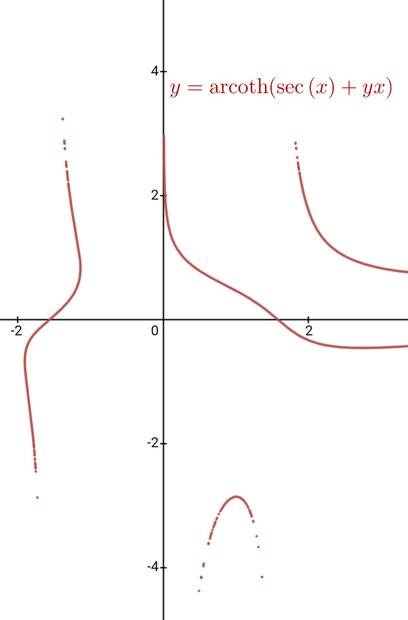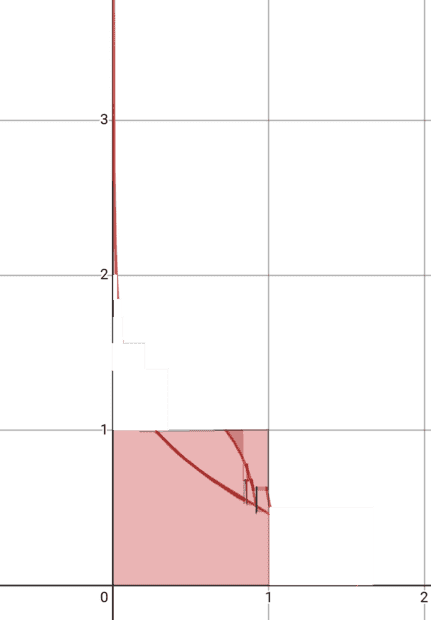# How to evaluate the enclosed area of this implicit curve?

• I
Saracen Rue
TL;DR Summary
How do you evaluate the area bound by an implicit curve when you can't rearrange for either variable or convert to a polar equation?
The implicit curve in question is ##y=\operatorname{arccoth}\left(\sec\left(x\right)+xy\right)##; a portion of the equations graph can be seen below:In particular, I'm interested in the area bound by the curve, the ##x##-axis and the ##y##-axis. As such, we can restrict the domain to ##[0, \frac{\pi}{2}]## and the range to ##[0,\infty)##

From what I can tell, there's no way to solve this equation for either ##y## or ##x##, so standard integration is out of the system. My next thought was to convert it to a poloar equation, which got me ##r\sin\left(\theta\right)=\operatorname{arccoth}\left(\sec\left(r\cos\left(\theta\right)\right)+r^{2}\cos\left(\theta\right)\sin\left(\theta\right)\right)##. However, this has the same issue of not being able to solve the equation for ##r##. After this, I did some Googling and I managed to find some reference to double integral methods on Maths Stack Exchange (the reference I used can be found here; in the answer that was marked as correct). However, I found the whole thing quite confusing and I'm not very confident I did it correctly. Regardless, I made an attempt: Firstly, I defined ##f(x,y)## like so:
$$f(x,y)= \begin{cases} 1 & \text{if }\operatorname{arccoth}\left(\sec\left(x\right)+xy\right)<y \\ 0 & \text{otherwise} \\ \end{cases}$$
I then proceeded to evaluate the double integeral like so and recieved the following result:
$$\int_{0}^{\frac{\pi}{2}}\int_{0}^{\frac{\pi}{2}}f\left(x,y\right)dy \text{ }dx \approx 1.46403068591$$

Okay so I have an actual way to get an answer now, but there are a few issues still.
1. I have no idea if I set my piecewise function up correctly; specifically for the domain on value for 1 - I just copied the general layout from another implicit curve area example and did my best to fit my equation too it.
2. I have no idea if my integration bounds are correct either - I assume they are but I also have a sinking suscipcsion that I have missed a step in determining the values to use here.
3. And finally, even if I have done everything else correctly, apparently this double integral method only generates approximate results and may not even be accurate.
So, to summarise, I want to know if my method of determining the area is correct, how accurate the result from my double integral is, and if it is at all possible to use a different method to get a more accurate result. Thank you all for taking the time to read this.

Homework Helper
Gold Member
Your y integration upper bound is wrong. It needs to be ##+\infty##.
I also think your inequality may be the wrong way around, so you are counting areas above the curve rather than below it.

I suggest the following approach, which works as long as the RHS of the equation (the part with the arccoth) has no discontinuities or missing points in the region you're integrating. You need to check that. There is a missing point at ##x = \pi/2## where the secant function disappears, but you can ignore that as long as you can confirm that the limit of the RHS as ##(x,y)\to(\pi/2,0)## is 0.

1. set a grid size h, say 0.001, but can make smaller for more accuracy
2. Find the sign of the RHS at ##(x,y)=(0,0)##
3. Run something like the following, in python-ish code:

Python:
h = 0.001
area = y  = 0
def g(x,y):
return arccoth(sec(x) + xy)
base_sign = sign(g(0,0) - 0)
x = 0
while sign(g(x,y)) == base_sign:
y = 0 # go to bottom of column
while sign(g(x,y)) == base_sign and (sign(g(x+h,y)) == base_sign or x > 0):
if sign(g(x+h, y+h)) == base_sign:
area += h * h # count the whole little square if sign hasn't changed at top-right corner
else:
area += 0.5 * h * h # count half the little square, since it crosses the line somewhere in the square
y += h # move up
x += h    # move right, to start next column

The idea is to do one column of little squares after another, moving left to right. Stop each column when you hit the curve. There's a bit of fiddling to deal with the curve's asymptote against the y axis.

An upper bound for the error in the area is approx (pi/2) * h / 2. Can you work out why?

Saracen Rue
Your y integration upper bound is wrong. It needs to be ##+\infty##.
I also think your inequality may be the wrong way around, so you are counting areas above the curve rather than below it.

I suggest the following approach, which works as long as the RHS of the equation (the part with the arccoth) has no discontinuities or missing points in the region you're integrating. You need to check that. There is a missing point at ##x = \pi/2## where the secant function disappears, but you can ignore that as long as you can confirm that the limit of the RHS as ##(x,y)\to(\pi/2,0)## is 0.

1. set a grid size h, say 0.001, but can make smaller for more accuracy
2. Find the sign of the RHS at ##(x,y)=(0,0)##
3. Run something like the following, in python-ish code:

Python:
h = 0.001
area = y  = 0
def g(x,y):
return arccoth(sec(x) + xy)
base_sign = sign(g(0,0) - 0)
x = 0
while sign(g(x,y)) == base_sign:
y = 0 # go to bottom of column
while sign(g(x,y)) == base_sign and (sign(g(x+h,y)) == base_sign or x > 0):
if sign(g(x+h, y+h)) == base_sign:
area += h * h # count the whole little square if sign hasn't changed at top-right corner
else:
area += 0.5 * h * h # count half the little square, since it crosses the line somewhere in the square
y += h # move up
x += h    # move right, to start next column

The idea is to do one column of little squares after another, moving left to right. Stop each column when you hit the curve. There's a bit of fiddling to deal with the curve's asymptote against the y axis.

An upper bound for the error in the area is approx (pi/2) * h / 2. Can you work out why?
Thank you for the reply, it helped a lot. I've amended the errors in the domain on the piecewise and on the integration bounds, so now I have:
$$f(x,y)= \begin{cases} 1 & \text{if }\operatorname{arccoth}\left(\sec\left(x\right)+xy\right)>y \\ 0 & \text{otherwise} \\ \end{cases}$$

$$\int_{0}^{\infty}\int_{0}^{\frac{\pi}{2}}f\left(x,y\right)dx \text{ }dy \approx 1.02554446649$$
So now the result of the double integral should at least be more accurate. To check if it's even remotely feasible, I took the graph I had and manually "cut" sections off to fit it all in the square like so:So it is looking fairly accurate from what I can tell. The darker shaded areas are effectively "double ups." Based of the estimation in the picture, the actual area under the graph seems to be slightly larger than the estimation from the double integral (probably in the 1.05 to 1.15 range), but we already knew that the double integral method was likely yo have a fairly substantial margin for error.

As for the missing point at ##x=\frac{\pi}{2}##, I don't believe it to be an issue due to the fact that the arccoth function still outputs a result of ##0## even for undefined values such as ##\sec{(\frac{\pi}{2})}## (I verified this using WolframAlpha).

As for the python code; I really do appreciate it but unfortunately I'm really not well versed in any proper coding like Pyhton (I can barely get Latex to work on sites like this 99% of the time). Would there be any other way to get a more accurate answer than the double integral via something like Desmos or Wolframalpha?

Oh, and as I side note, I did attempt to get WolframAlpha to calculate the double integral as I assume it'd be able to generate a more accurate result than Desmos did, but Wolfram kept running out of processing time as I only have the free version. If anyone reading this has a pro version of WolframAlpha (or a Mathamatica subscription) I'd really appreciate it if you could give the double integral a go on there: the WolframAlpha Langauge input string is: Integrate[{Piecewise[{{1,ArcCoth[x y + Sec[x]] > y}}]},{x ,0,π/2},{y,0,∞}]